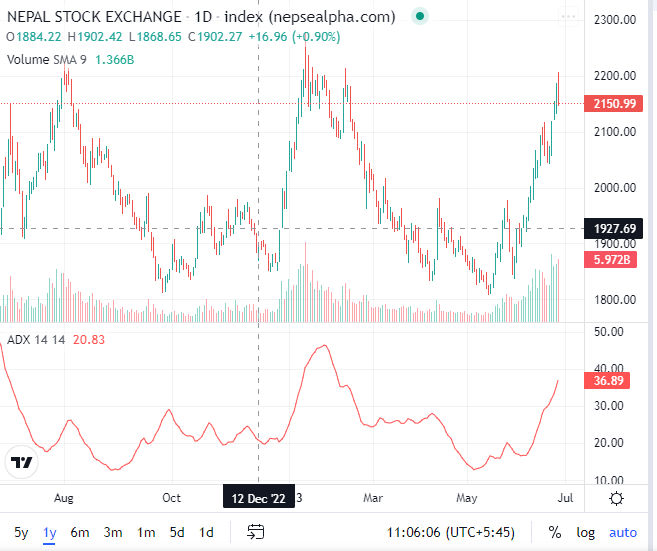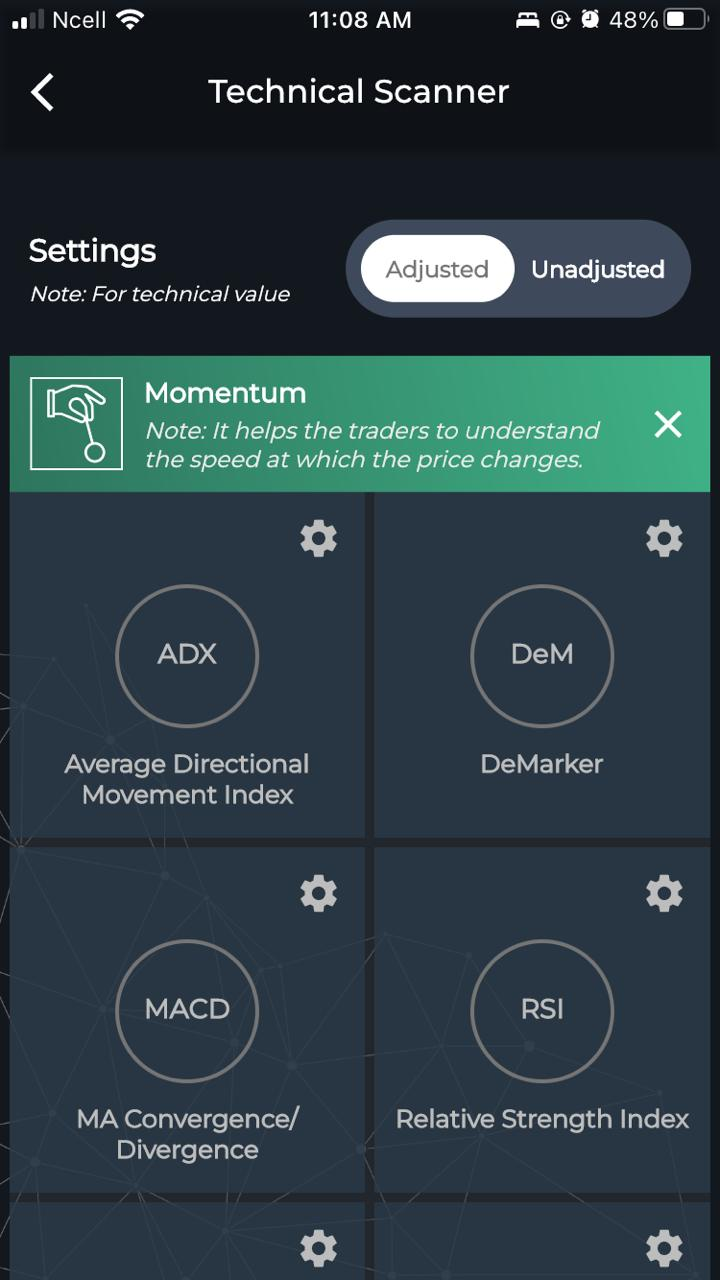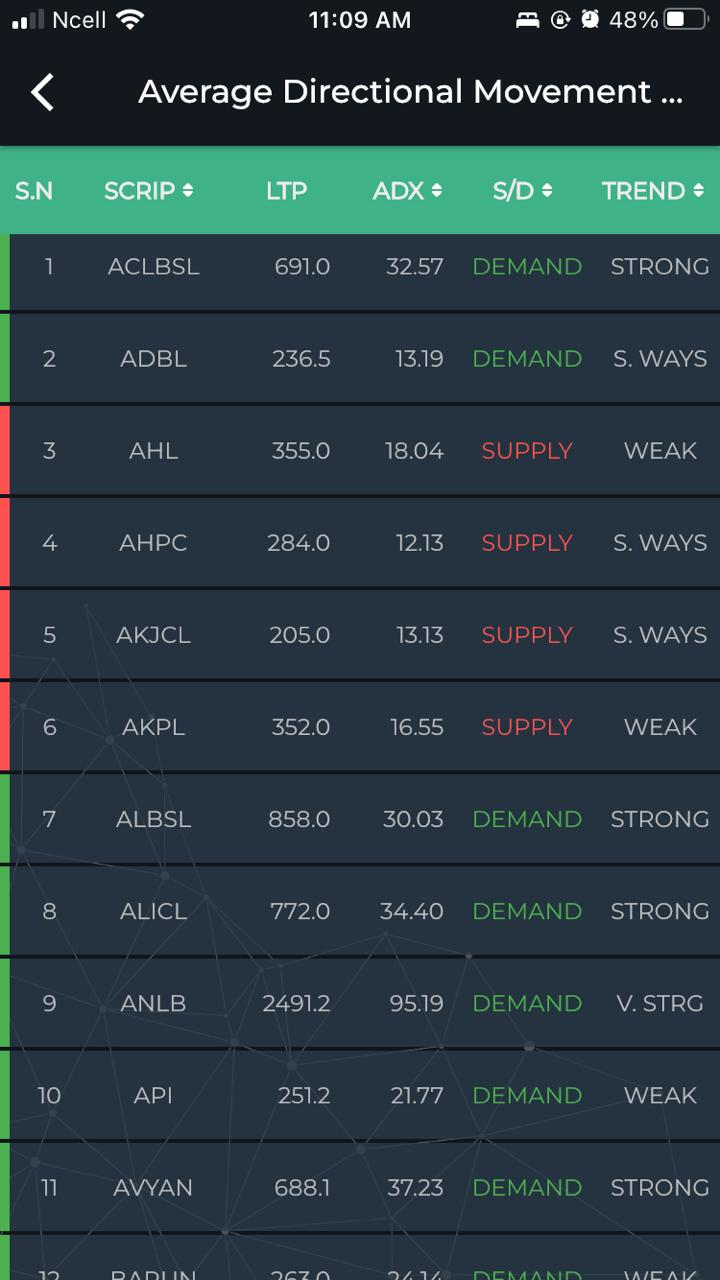The average directional index (ADX) is a technical analysis indicator used by some traders to determine the strength of a trend. The trend can be either up or down, and this is shown by two accompanying indicators, the negative directional indicator (-DI) and the positive directional indicator (+DI). Therefore, the ADX commonly includes three separate lines. These are used to help assess whether a trade should be taken long or short, or if a trade should be taken at all.

• The ADX makes use of a positive (+DI) and negative (-DI) directional indicator in addition to the trendline.
• The Average Directional Index (ADX) is in turn derived from the smoothed averages of the difference between +DI and -DI; it measures the strength of the trend (regardless of direction) over time## The Average Directional Index (ADX) Formula :

The ADX requires a sequence of calculations due to the multiple lines in the indicator.

+DI=(Smoothed +DMATR )×100

-DI=(Smoothed -DMATR )×100

DX=(∣+DI−-DI∣∣+DI+-DI∣)×100

where:

+DM (Directional Movement)=Current High−PH

PH=Previous High

-DM=Previous Low−Current Low

Smoothed +/-DM=∑�=114DM−(∑�=114DM14)+CDM

CDM=Current DM

ATR=Average True Range

### Calculating the Average Directional Movement Index (ADX)

1. Calculate +DM, -DM, and the true range (TR) for each period. Fourteen periods are typically used.
2. +DM = current high - previous high.
3. -DM = previous low - current low.
4. Use +DM when current high - previous high > previous low - current low. Use -DM when previous low - current low > current high - previous high.
5. TR is the greater of the current high - current low, current high - previous close, or current low - previous close.
6. Smooth the 14-period averages of +DM, -DM, and TR—the TR formula is below. Insert the -DM and +DM values to calculate the smoothed averages of those.
7. First 14TR = sum of first 14 TR readings.
8. Next 14TR value = first 14TR - (prior 14TR/14) + current TR.
9. Next, divide the smoothed +DM value by the smoothed TR value to get +DI. Multiply by 100.
10. Divide the smoothed -DM value by the smoothed TR value to get -DI. Multiply by 100.
11. The directional movement index (DMI) is +DI minus -DI, divided by the sum of +DI and -DI (all absolute values). Multiply by 100.
12. To get the ADX, continue to calculate DX values for at least 14 periods. Then, smooth the results to get ADX.
13. First ADX = sum 14 periods of DX / 14.
14. After that, ADX = ((prior ADX * 13) + current DX) / 14.

You can scan ADX in smart karobaar app with following process :

## 1) At first you have to open smart karobaar app

2) Then tap on technical scanner

3) After that you have to tap on ADX under momentum

4) At last you can scan ADX .